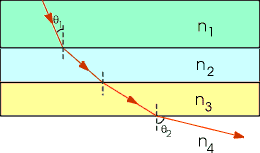# Multiplayer Refraction, Incident Angle

## Homework Statement

The figure below shows the path of a beam of light through several layers (n1 = 1.58, n2 = 1.42, n3 = 1.20 and n4 = 1.00) of different indices of refraction.
https://loncapa2.physics.sc.edu/res/brookscole/serway/College_Physics_7ed/Chap22/graphics/serw2244.gif

a) If θ1 = 30.3 deg, what is the angle, θ2, of the emerging beam? (5.29×10^1 deg)

b) What must the incident angle, θ1, be in order to have total internal reflection at the surface between the n3 = 1.20 medium and the n4 = 1.00 medium?

## Homework Equations

For part a)
n1sin(theta1)=n2sin(alpha)
n2sin(alpha)=n3sin(beta)
n3sin(beta)=n4sin(theta2)

For part b) I'm not too sure.

## The Attempt at a Solution

For part a)
n1sin(theta1)=n2sin(alpha)
(1.58)sin(30.3)=(1.42)sin(alpha)
alpha=34.15099

n2sin(alpha)=n3sin(beta)
(1.42)sin(34.15099)=(1.20)sin(beta)
beta=41.628239

n3sin(beta)=n4sin(theta2)
(1.20)sin(41.628)=(1)sin(theta2)
theta2=52.90 deg ---> this is the correct answer
(the formulas can also be simplified to... theta2=arcsin(n1sin(theta1)/n4)

For part b)
This is the part I"m having difficulties with.
(1.20)sin(theta)=(1.00)sin(90)
sin(theta)=(1.00)/(1.20)
theta=56.44269 deg
I know that this isn't the answer. Am I right in connecting the total internal reflection with sin(90)? Is the critical angle equivalent to the incident angle...are they the same thing?That angle that you found is the angle of incidence in the n3 medium. I think the question asks you for θ1, which is the incidence angle in the n1 medium. If you use Snells law to find θ1, that might be what you're looking for.

•rlc
(n3)sin(beta)=(n4)sin(90)
(1.20)sin(beta)=(1.00)sin90
beta=56.44

(n2)sin(alpha)=(n3)sin(beta)
(1.42)sin(alpha)=(1.20)sin(56.44)
alpha=44.7669

(n1)sin(theta1)=(n2)sin(alpha)
(1.58)sin(theta1)=(1.42)sin(44.7669)
theta1=39.26 deg